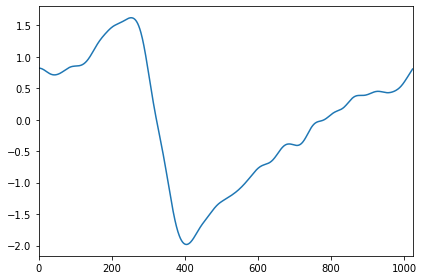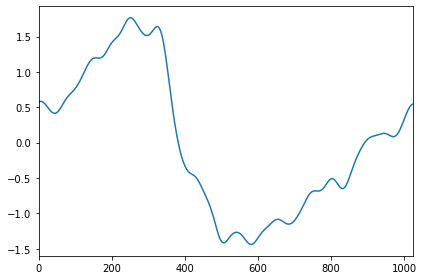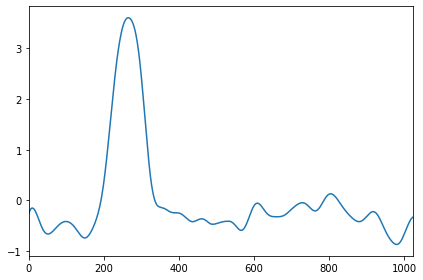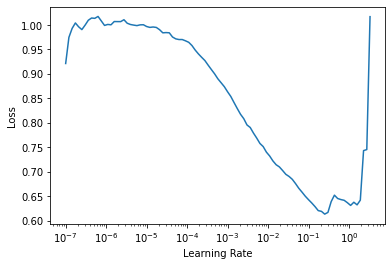Lesson Video:

This article is also a Jupyter Notebook available to be run from the top down. There will be code snippets that you can then run in any environment.

Below are the versions of fastai, fastcore, wwf, and tsai currently running at the time of writing this:

• fastai: 2.1.10
• fastcore: 1.3.13
• wwf: 0.0.8
• tsai: 0.2.12

This module was built by Ignacio Oguiza among others, see this megathread for the discussion. The essential goal here is we can use arrays of any dimension instead of just 1 (such as tabular) or 3 (such as images).

• Note, this notebook was heavily influenced from his tutorial notebook here

Now let's grab what we need:

from fastai.tabular.all import *
from tsai.all import *

For our data we'll be utilizing the UCR repository which has 128 univariate and 30 multivariate datasets. In the framework we can quickly grab any dataset we want:

name = 'StarLightCurves'

We can now grab our train and validation by calling get_UCR_data:

X_train, y_train, X_valid, y_valid = get_UCR_data(name, verbose=True, on_disk=True)
Dataset: StarLightCurves
decompressing data...
...data decompressed
X_train: (1000, 1, 1024)
y_train: (1000,)
X_valid: (8236, 1, 1024)
y_valid: (8236,)

Since data is already split between train and test in the UCR dataset, we are going to merge them and create some indices to split them in the same sets. To save on memory, he figured out a way to utilized the numpy arrays via your disk. We'll do so as follows:

X = np.concatenate((X_train, X_valid))
y = np.concatenate((y_train, y_valid))
np.save('./data/UCR/StarLightCurves/X.npy', X)
np.save('./data/UCR/StarLightCurves/y.npy', y)
del X, y

Now we can load them back in and make our splits:

splits = (L(np.arange(len(X_train)), use_list=True),
L(np.arange(len(X_train), len(X)), use_list=True))
splits
((#1000) [0,1,2,3,4,5,6,7,8,9...],
(#8236) [1000,1001,1002,1003,1004,1005,1006,1007,1008,1009...])

Since we used memmap the data is being directly read from memory. Now to make and use your own data they need to be in a three dimentional array with a format of:

• Samples
• Variables
• Length (or timesteps)

To use this we have a special TSTensor built to handle such data:

t = TSTensor(X)
/usr/local/lib/python3.6/dist-packages/fastai/torch_core.py:117: UserWarning: The given NumPy array is not writeable, and PyTorch does not support non-writeable tensors. This means you can write to the underlying (supposedly non-writeable) NumPy array using the tensor. You may want to copy the array to protect its data or make it writeable before converting it to a tensor. This type of warning will be suppressed for the rest of this program. (Triggered internally at  /pytorch/torch/csrc/utils/tensor_numpy.cpp:141.)
t
TSTensor(samples:9236, vars:1, len:1024)

So here we can see we had 60 samples with one variable and an overall time length of 570 for each

We can directly use this in our DataBlock API too! With a TSTensorBlock:

splitter = IndexSplitter(splits)
getters = [ItemGetter(0), ItemGetter(1)]
dblock = DataBlock(blocks=(TSTensorBlock, CategoryBlock),
getters=getters,
splitter=splitter)

Next we make a call to itemify which zips up our x's and our y's

src = itemify(X, y)

And now we can make our DataLoaders:

dls.show_batch(max_n=3)And there's our data! Now are we still on disk? Yes

dls.dataset
(memmap([[0.5373029 , 0.53110296, 0.52850294, ..., 0.52640295, 0.51950294,
0.51140296]], dtype=float32), TensorCategory(2))

Training our Model

The particular model we're using is the Inception Time model. To do so we need the number of input classes and our number of variables:

dls.c
3
inp_vars = dls.dataset.shape[-2]
inp_vars
1
net = InceptionTime(inp_vars, dls.c)
learn = Learner(dls, net, loss_func=CrossEntropyLossFlat(), metrics=accuracy, opt_func=ranger)
learn.lr_find()
SuggestedLRs(lr_min=0.025118863582611083, lr_steep=0.002511886414140463)And now we can fit!

learn.fit_flat_cos(10, 0.025)
epoch train_loss valid_loss accuracy time
0 0.539881 1.406547 0.641938 00:07
1 0.433147 0.335748 0.851506 00:07
2 0.373533 2.214681 0.559009 00:07
3 0.325850 0.599999 0.852477 00:07
4 0.295900 0.382158 0.837785 00:07
5 0.270379 0.178628 0.942205 00:07
6 0.252287 1.122112 0.765542 00:07
7 0.230175 0.151228 0.949247 00:07
8 0.200199 0.110600 0.972195 00:07
9 0.172292 0.106513 0.973045 00:07